Courses

# Test: Practical Geometry - 3

## 20 Questions MCQ Test Mathematics (Maths) Class 6 | Test: Practical Geometry - 3

Description
This mock test of Test: Practical Geometry - 3 for Class 6 helps you for every Class 6 entrance exam. This contains 20 Multiple Choice Questions for Class 6 Test: Practical Geometry - 3 (mcq) to study with solutions a complete question bank. The solved questions answers in this Test: Practical Geometry - 3 quiz give you a good mix of easy questions and tough questions. Class 6 students definitely take this Test: Practical Geometry - 3 exercise for a better result in the exam. You can find other Test: Practical Geometry - 3 extra questions, long questions & short questions for Class 6 on EduRev as well by searching above.
QUESTION: 1

Solution:
QUESTION: 2

Solution:
QUESTION: 3

### What do you call two lines intersecting at a point?

Solution:
QUESTION: 4

An angle of 15° is drawn using a pair of compasses and a ruler. How is it done?

Solution:
QUESTION: 5

Which of the following is an angle that can be constructed using compasses and a ruler?

Solution:
QUESTION: 6

How do you draw a 90∘ angle?

Solution:
QUESTION: 7

An angle ∠XYZ = 75 is bisected by an angular bisector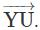. Then what is the measure of∠UYZ?

Solution:
QUESTION: 8

A line segment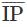is bisected at T. Which of the following equals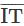?

Solution:
QUESTION: 9

Which of the following is done to draw an angle of 150 using compasses and a ruler?

Solution:
QUESTION: 10

Two lines are said to be perpendicular to each other when they meet at ____angle.

Solution:
QUESTION: 11

A perpendicular is drawn to a line segment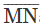at N using a protractor and a point P is marked on it. Which of the following is true?

Solution:
QUESTION: 12

If two lines have only one point in common, what are they called?

Solution:

Intersecting lines have only one point in common.

QUESTION: 13

For which of the following are the rails of a railway line an example?

Solution:

The rails of a railway line are an example of parallel lines.

QUESTION: 14

Identify the one with no definite length.

Solution: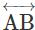has no definite length.

QUESTION: 15

A maths teacher asked his students to draw a pair of parallel lines. Which instrument(s) are the students most likely to use?

Solution:

The lines drawn using the two edges of a ruler are parallel. Also a ruler and a setsquare can be used to draw a pair of parallel lines.

QUESTION: 16

Identify the pair of parallel lines.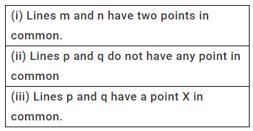Solution:

Parallel lines do not have any point in common.

QUESTION: 17

A few lines in a plane have a point in common. What type of lines can they be?

Solution:

If the lines are only two, then they are intersecting lines. If there are more than two lines, then they are concurrent lines.

QUESTION: 18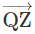is the bisector of ∠PQZ = ∠PQR. Which of the following is true?

Solution:bisects ∠PQR  (Given)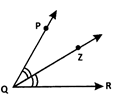Thus. ∠PQZ = ∠ZQR = 1/2 ∠PQR

QUESTION: 19

The point M on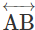is such that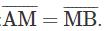Which of the following is a false statement?

Solution:

M is a point onsuch thatThen M is the midpoint of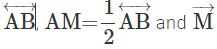bisectsare true.
Hence the only false statement is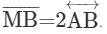QUESTION: 20

Lines a, b, p, q, m, n and x have a point P common to all of them. What is the name of P?

Solution:

A point common to multiple lines is called a point of concurrence as the lines are concurrent lines.